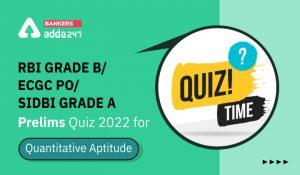Latest Banking jobs   »   Quantitative Aptitude Quiz 14 June- Daily...

# Quantitative Aptitude Quiz 14 June- Daily Quantitative Aptitude Quiz for SBI PO Prelims 2020

Adda 247 will provide you daily Quantitative Aptitude Mock of “SBI PO Prelims. In today’s Quantitative Aptitude Mock, we have Quadratic Inequalities . Stay tuned with bankersadda to get  Quantitative Aptitude Mock and boost your preparation for the upcoming bank exam. You can also prepare with the study plan for SBI PO available on the bankersadda website.Directions (1-15): In the following two equations questions numbered (I) and (II) are given. You have to solve both equations and Give answer

Q1.(a) If x > y

(b) If x ≥ y

(c) If y > x

(d) If y ≥ x

(e) If x = y or no relation can be established

Q2.(a) If x > y

(b) If x ≥ y

(c) If y > x

(d) If y ≥ x

(e) If x = y or no relation can be established

Q3.(a) If x > y

(b) If x ≥ y

(c) If y > x

(d) If y ≥ x

(e) If x = y or no relation can be established

Q4.(a) If x > y

(b) If x ≥ y

(c) If y > x

(d) If y ≥ x

(e) If x = y or no relation can be established

Q5.(a) If x > y

(b) If x ≥ y

(c) If y > x

(d) If y ≥ x

(e) If x = y or no relation can be established

To attempt the complete quiz refer to the links given below:

Practice with Crash Course and Online Test Series for SBI PO Prelims:

#### Congratulations!Incorrect details? Fill the form again here

•Quantitative Aptitude Quiz For IDBI AM/E...
•Quantitative Aptitude Quiz For IDBI AM/E...
•Quantitative Aptitude Quiz For IDBI AM/E...
•Quantitative Aptitude Quiz For SBI Clerk...
•Quantitative Aptitude Quiz For RBI Grade...
•Quantitative Aptitude Quiz For SBI Clerk...